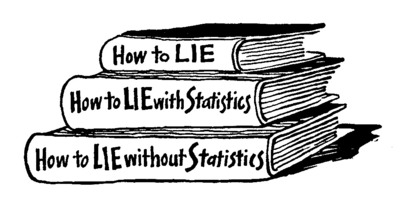Intro Research PhD study R codes Teaching Extra

## NMST434 Modern statistical methods (official information)

Exercise class: Monday 14:00 - 15:30 K2 (S. Nagy)

Assumed knowledge:

It is assumed that the students have already a very solid knowledge of statistics and probability theory. This is covered by the following courses Mathematical Statistics 1 and 2 (NMSA331 and NMSA332), Probability Theory 1 (NMSA333) and Linear regression (NMSA407).

The student who have not passed these courses (e.g. students coming within the Erasmus+ programme) should check if they are familiar with the theory covered in:
Mukhopadhyay, N. (2000). Probability and statistical inference. CRC Press (the whole book except for Chapters 10 and 13);
Khuri, A. I. (2009). Linear model methodology. Chapman and Hall/CRC (Chapters 1 - 6).

(Assumed) content of the course:
• Asymptotic methods - Delta Theorem, Moment estimators
• Theory of maximum likelihood
• Profile, conditional and marginal likelihood
• M-estimators and Z-estimators, Robust estimation
• Bootstrap
• Quantile regression
• EM-algorithm
• Methods for missing data
• Kernel density estimation
• Kernel nonparametric regression

Lecture material and actual information